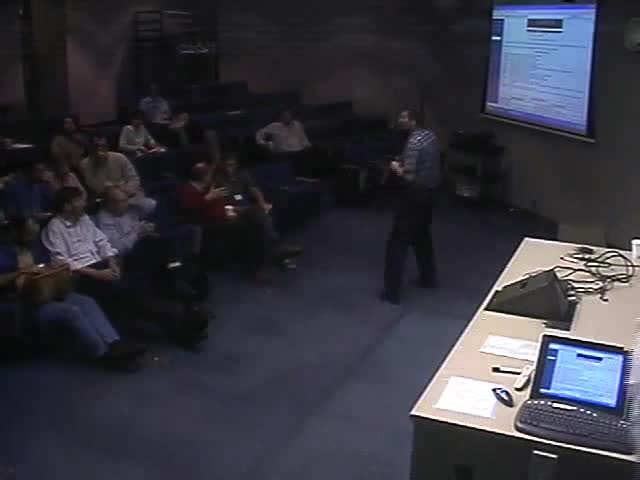### Videos

#### Solution of Integral and Differential Equations using Algebraic Splines

##### Presenter
September 16, 2006##### Abstract
This two part tutorial shall introduce you to algorithmic algebraic geometry methods of manipulating algebraic (polynomial) splines necessary for the solution of multivariate integral and partial differential equations emanating from science and engineering applications. In the first part, we shall discuss well known (classical) algorithms for modeling the structure and energetics of physical domains (molecules to airplanes to oil fields), at multiple scales, with implicit algebraic splines (A-patches), and their rational parametric splines (NURBS) approximations. Algebraic geometry methods include computing global and local parameterizations using Newton factorization and Hensel lifting, computation of adjoints by interpolating through singularities, and low degree curve and surface intersections via Resultants and/or Groebner Basis calculations. The second part shall focus on more current research and attempts at using algebraic geometry methods for faster and more accurate calculations of multivariate definite integrals of algebraic functions arising from the solution of polarization energetics and forces (Generalized Born, Poisson Boltzmann) and Green function solutions of two-phase Stokesian flows. These algebraic geometry methods include the use of ideal theory and solutions of polynomial systems of equations for more accurate cubature formulas and their faster evaluation.